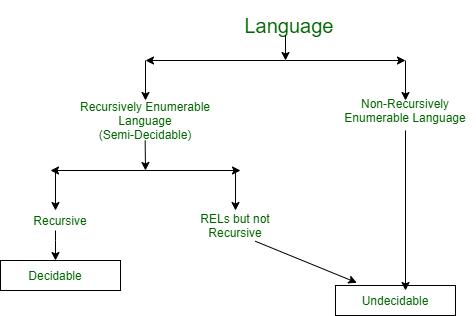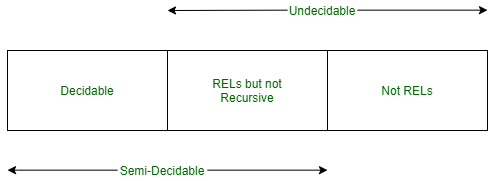# Decidability, Semi-Decidability, and Undecidability in TOC

• Difficulty Level : Expert
• Last Updated : 08 Apr, 2021

We know about Decidable, Semi-decidable, and Undecidable problems and in this article, we will briefly define these problems and provide the most commonly asked questions on these problems and classify them accordingly.

Attention reader! Don’t stop learning now.  Practice GATE exam well before the actual exam with the subject-wise and overall quizzes available in GATE Test Series Course.

Learn all GATE CS concepts with Free Live Classes on our youtube channel.

Introduction :
Identifying the type of language is a very frequently asked question in Gate and other exams. However, there are some problems which are frequently asked and here in this article we shall discuss all those problems. We shall also solve few examples in this article to make the topic more clear.Decidable Problems :
The decidable problems are those problems for which there exists a corresponding Turing machine that halts on every input with an answer- yes (accepting) or no (rejecting).  It is also called Turing Decidable.

Semi-Decidable Problems :
Semi-Decidable problems are those problems for which a Turing machine halts on the input accepted by it but can either loop forever or halt on the input which is rejected by the Turing Machine. It is also called Turing Recognizable problems.

Undecidable Problems :
Undecidable problems are those problems for which there exists no Turing machine which will always halt an infinite amount of time to give an answer as ‘yes’ or ‘no’. An undecidable problem has no algorithm to determine the answer for a given input. It can be partially decidable but never decidable. They are also known as Non-Recursively Enumerable Language.Classification Table:
Now we will classify most commonly asked problems as Decidable, Semi-decidable and Undecidable. Here in the tables below, D means Decidable, SD means Semi-Decidable and NR means Not-Recursively Enumerable. Undecidable can be either Semi-Decidable or Not-Recursively Enumerable language.

Decidability Classification Table :
Another table is as follows.

Examples :
Here, we will discuss some examples for better understanding as follows.

Example-1 :
{TM | No. of states in TM=2. }
Solution –
Decidable as for this we have to find no. of nodes in the graph.

Example-2 :
TM halts after 100 moves.
Solution –
Decidable as we have logic for both ‘yes’ or ‘no’.

```Yes : No string halts within 100 moves. (Check up to 100 length string).
No  : At least one string halts within 100 moves.```

Example-3 :
TM halts on w after 100 moves.
Solution –
Semi-Decidable as we have logic for only ‘yes’ and no logic for ‘no’.

```Yes : Halts after 100 moves has a logic.
No  : Doesn't halt after 100 moves have no logic as can go into an infinite loop or never halt.```

Example-4 :
TM reaches state q.
Solution –
Semi-Decidable as we have logic for only ‘yes’ and no logic for ‘no’.

```Yes : We have logic for yes as TM reaches state q we will come to know.
No  : No logic for no as TM can never reach state q or can fall into
an infinite loop before reaching q and will never come to know.```

Example-5 :
Consider when given grammar generates Context Free Language.
Solution –
Undecidable as we have no logic for ‘Yes’ — that the given grammar generates CFL.

My Personal Notes arrow_drop_up